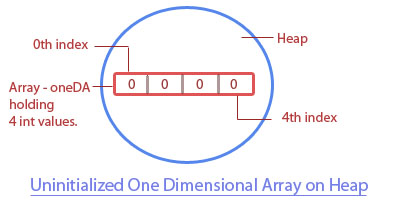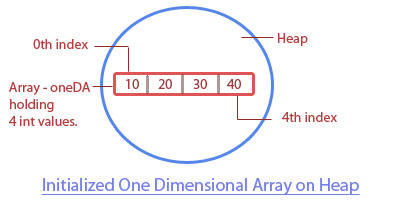< Prev
Next >

# C# Array

Like all programming languages, the C# language also allows us to hold a set of similar data types together, through its feature known as array. An array allows us to hold multiple values of the same type.

These multiple values held by an array could be -
• Primitive values of the same type , or
• Object references of the same type.

We will explain how to create arrays holding these two different types of values in the upcoming section. But before that you should know that once an array is created, its size to hold multiple values cannot be modified, hence an array is static in nature.

## Types of array -

• One-dimensional array(1-D) is a single array that holds multiple values of the same type, i.e. the same type of primitive values or object references.

• Two-dimensional array(2-D) is an array containing multiple arrays within it, where all of these multiple arrays are holding values of the same type i.e. the same type of primitive values or the same type of object references.

## Note:

An Array is an object which is stored on the Heap memory.

We will understand about the one-dimensional array(1-D) in three separate parts -
• Array declaration
• Array construction
• Array initialization
Let's understand the steps involved in the declaration, construction, and initialization of a one-dimensional(1-D) array.

## Declaration of a 1-D array

The general form of only declaring a one dimensional array is :

``type[] array-name;``
where:
• type is the type of elements this array will hold, followed by an empty pair of square brackets [ ]
• array-name, is the name of the array.

Let us show you an example in which we are going to declare a 1-D array that is going to hold primitive int values.

``````//C# Example of declaring an array

using System;

class A
{
public static void Main()
{
int[] array; //declaration of a 1-D array
}
}``````

We have only declared a one-dimensional array which doesn't exist on the heap yet.

## Construction of a 1-D array

The general form of declaring and constructing a one dimensional array is :

``type[] array-name = new type[size]``
where:
• type is the type of elements this array will hold, followed by a empty pair of square brackets [ ].
• array-name, is the name of the array.
• new is the keyword using which an array is constructed and gets the memory allocation.
• size is the size of the array i.e. total number of elements it will hold

Let us show you an example in which an array is constructed on the heap when its size is mentioned at the same time of its creation by using the new keyword.

``````//C# Example of constructing a 2D array

using System;

class A
{
public static void Main()
{
int[] array= new int; //Array object is constructed on the heap with size to hold 4 int values.
}
}```
```In the example, the int array is constructed on the heap by using the new keyword, but its 4 int values aren't manually initialized by us, therefore, by default, this array has automatically initialized itself with 4 zero values in it. This array is large enough to hold 4 elements of type int and it is referenced by the reference variable array.

Note: If we don't initialize an array that holds at the time of its construction, it is automatically initialized with a default value:
• An array holding any numeric primitive type values will be automatically initialized to zero(0), and,
• An array holding object references will be automatically initialized to null.
Please look at the table to know more -

Array type Default value
char '\u0000'
byte 0
short 0
int 0
long 0
float 0
double 0
bool false
String null
Object null

## Initialization of a 1-D array

Array elements are stored using their index position in an array, with the first element stored at index zero and so on.

``````//C# Example of initializing an array

using System;

class A
{
public static void Main()
{
//Array object is constructed on the heap when its size is mentioned at its creation.
int[] oneDA = new int;

//storing 10 at index 0 in array
oneDA = 10;

//storing 20 at index 1 in array
oneDA = 20;

//storing 30 at index 2 in array
oneDA = 30;

//storing 40 at index 3 in array
oneDA = 40;
}
}```
```• The same array could also be constructed and initialized in a single line, without using the new keyword:

``int[] oneDA = {10, 20, 30, 40};``

## Reading of a 1-D array using for loop

We can read the elements of an array using their index position in the array. For example - to read an element at an index 2 in an array named arr is as simple as -

``````Console.WriteLine(arr);	//Reading 2nd index of this array gives us a value, 30
``````

But imagine when we have to read an array of 1000 elements, does it mean that we have to type in 1000 lines of code to read each element from its index? No, in order to easily read through all the elements of an array(irrespective of its size), we may use the Length property of an array within the for-loop.

The Length property tells us the total number of elements an array can hold, which is one more than the last index position at which an element is stored in an array.

For example - As you know that in an array, elements are stored starting at an index zero, hence if the length of an array is 5, the first element is stored at an index position 0 and the last element is stored at an index position 4.

``````//C# Example of using the Length property of an array

using System;

class A
{
public static void Main()
{
//Array object is constructed on the heap when its size is mentioned at its creation.
char[] array = new char;

array = 'a';
array = 'b';
array = 'c';
array = 'd';

//Accessing the array elements by using its Length property in the for-loop
for(int i=0; i<array.Length; i++)
{
Console.WriteLine(array[i]);
}
}
}```
```

## Output -

``````a
b
c
d``````

## 1-D Array holding object references

So far, we read about one-dimensional array holding primitive values but an array can also be used to store object references (some people may call storing objects but in reality, only references of objects are stored).``````//C# Example of array holding object references of the same type

using System;

class A
{
public static void Main()
{
//Array holding 4 object references of type, A.
A[] array= new A;

//Creating 4 object of type A
A ob1 = new A();
A ob2 = new A();
A ob3 = new A();
A ob4 = new A();

//Assigning reference of the first object to the first array element
//with index position 0
array = ob1;

//Assigning reference of the second object to the second array element
//with index position 1
array = ob2;

//Assigning reference of the third object to the third array element
//with index position 2
array = ob3;

//Assigning reference of the fourth object to the fourth array element
//with index position 3
array = ob4;

//length of an array.
Console.WriteLine("Length of the array holding object references: "+ array.Length);

//Accessing the array elements by using its Length property in for-loop
for(int i=0; i<array.Length; i++)
{
Console.WriteLine(array[i]);
}
}
}``````

## Output-

``````Length of the array holding object references: 4
A
A
A
A``````

Unlike Java, where printing an object of a class prints a hexadecimal code, the C# language only displays the name of the class the object belongs to, therefore, in our last example, only the name of the class is displayed when the contents of an array holding object references are displayed.

## An array holding object references will be automatically initialized to null

``````//C# Example of using the Length property of an array

using System;

class A
{
public static void Main()
{
//An array object to hold 4 objects of type A is constructed and initialized on the heap
//The elements of this array will be automatically initialized to null
A[] array = new A;

//Accessing the array elements by using its Length property in the for-loop
for(int i=0; i<array.Length; i++)
{
if(array[i] == null)
Console.WriteLine(array[i] + "A null element");
}
}
}``````

## Output:

``````A null element
A null element
A null element
A null element``````

As you can see in the output of our last example, an array object to hold 4 objects of type A is constructed and initialized on the heap. The elements of this array will be automatically initialized to null, which means that each reference points to no value i.e. not initialized and therefore, nothing is printed when the null is printed.

Please subscribe to our social media channels for daily updates.